Core Java - Interview Questions and Answers for '==' | Search Java Interview Question - javasearch.buggybread.com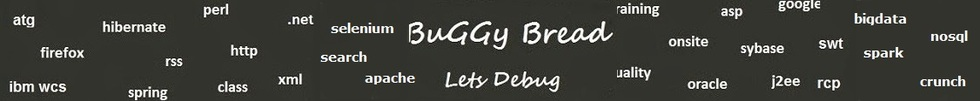# Search Interview QuestionsMore than 3000 questions in repository.There are more than 900 unanswered questions.Have a video suggestion.
Click Correct / Improve and please let us know.
Label / Company      Label / Company / TextSubmit Question

Core Java - Interview Questions and Answers for '==' - 10 question(s) found - Order By Newest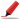Very frequently asked. Among first few questions in almost all interviews. Among Top 5 frequently asked questions. Frequently asked in Indian service companies (HCL,TCS,Infosys,Capgemini etc based on multiple feedback )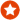Q1. Difference between == and .equals() ?Core Java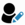Anonymous
Ans. "equals" is the method of object class which is supposed to be overridden to check object equality, whereas "==" operator evaluate to see if the object handlers on the left and right are pointing to the same object in memory.

x.equals(y) means the references x and y are holding objects that are equal. x==y means that the references x and y have same object.

Sample code:

String x = new String("str");
String y = new String("str");

System.out.println(x == y); // prints false
System.out.println(x.equals(y)); // prints true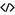Sample Code for equalsHelp us improve. Please let us know the company, where you were asked this question :LikeDiscussCorrect / Improvejava   string comparison   string   object class   ==    equals   object equality  operator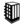Asked in 292 Companies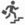basic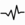frequent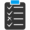Try 6 Question(s) TestQ2. What will this code print ?

String a = new String ("TEST");
String b = new String ("TEST");
if(a == b) {
System.out.println ("TRUE");
} else {
System.out.println ("FALSE");
}
Core JavaAnonymous
Ans. FALSE. == operator compares object references, a and b are references to two different objects, hence the FALSE. .equals method is used to compare string object content.Help us improve. Please let us know the company, where you were asked this question :LikeDiscussCorrect / Improvestring   string class   java   ==   object references   codingAsked in 2 CompaniesbasicfrequentTry 1 Question(s) TestQ3. What will be the output of following Code ?

public static void main(String[] args) {
String s2 = "I am unique!";
String s5 = "I am unique!";

System.out.println(s2 == s5);
}
}
Core JavaAdmin info@buggybread.com
Ans. true, due to String Pool, both will point to a same String object.Help us improve. Please let us know the company, where you were asked this question :LikeDiscussCorrect / Improvejava   code   coding   tricky questions   interesting questions   string   string pool   .equal   ==intermediatefrequentQ4. What is wrong with the following if statement ?

if(x==y && x.equals(y) {
}
Core Java2016-07-28 13:45:21Ans. x==y means that both references have same type and are pointing to same memory location and hence would always mean that they have same value.

x.equals(y) is not required in this case.Help us improve. Please let us know the company, where you were asked this question :LikeDiscussCorrect / Improveif statement  control statements  == and equals  ==  .equals   code optimizationTry 2 Question(s) TestQ5. What is wrong with the following if statement ?

if(x==y || x.equals(y) {
}
Core Java2016-07-28 13:48:23Ans. if x==y turns out to be true x.equals(y) will be true too. If x.equals(y) could be true even if x==y is true or not.

So the only possible outcomes are

1 || 1 = 1
0 || 1 = 1
0 || 0 = 0

i.e the outcome of x.equals(y)

check for x==y is not required in this if statement.Help us improve. Please let us know the company, where you were asked this question :LikeDiscussCorrect / Improveif statement  control statements  == and equals  ==  .equals   code optimizationTry 1 Question(s) TestQ6. What is the difference between = and == in Java ?Core Java2016-09-02 08:12:45Ans. = is the assignment operator that assigns the result of the expression on the right to the variable on the left, whereas

== is the operator to check object equality to see if the reference on left and right are pointing to the same object. For primitive types, its used to check if both variables holds the same value.Help us improve. Please let us know the company, where you were asked this question :LikeDiscussCorrect / Improve=  ==  assignment operator  object equality  difference betweenBasicQ7. How is == operator different for objects and primitive types ? Core Java2016-09-02 08:14:44Ans. For objects or references, == operator check if the reference on left and right points to the same object.

For primitive types or variables, == operator check if the variable on left and right holds the same value.Help us improve. Please let us know the company, where you were asked this question :LikeDiscussCorrect / Improve==  object equality  operatorBasicQ8. What will be the output of following code

Integer x = 1;
Integer y = 2;
System.out.println(x == y);

What if you change 1 to "1" and Integer to String?
Core Java2017-05-07 19:30:13Ans. It will print "true" with integers as well as strings. The reason is "Integer constant pool" and "String pool"

String pool maintains pool of string literals. When a string literal is used for the first time, a new string object is created and is added to the pool. Upon it's subsequent usage , the reference for the same object is returned. Similarly java uses integer constant pool.Help us improve. Please let us know the company, where you were asked this question :LikeDiscussCorrect / Improvestring pool  object equality  ==Q9. What will be the output of following code

class TestMain {
public static void main(String[] args) {
String s1 = "ravi";
String s2 = new String("ravi");
Integer i = 10;
Integer a1 = new Integer("10");
System.out.println(s1 == s1);
System.out.println(i == a1);
}
}
Core Java2017-07-17 08:07:19Ans. false
falseHelp us improve. Please let us know the company, where you were asked this question :LikeDiscussCorrect / Improveobject equality  ==  coding  codeAsked in 1 CompaniesQ10. Difference between == and === ?JavaScript2018-07-04 16:16:18Ans. == compares values === is used in scripting languages for comparing two values as well as there data tpe. like in js,php.Help us improve. Please let us know the company, where you were asked this question :LikeDiscussCorrect / Improve==  ===  == vs ===Asked in 13 CompaniesbasicfrequentQ11. Which of the following is true for == operator ? Core Java
a. For primitives, == checks if the variables on left and right have same data type
b. For primitives, == checks if the variables on left and right have same value
c. For Objects, == checks if the references on left and right have same data type
d. For Objects, == checks if the references on left and right have same value

Ans.b. For primitives, == checks if the variables on left and right have same value

## Help us and Others Improve. Please let us know the questions asked in any of your previous interview.

Any input from you will be highly appreciated and It will unlock the application for 10 more requests.